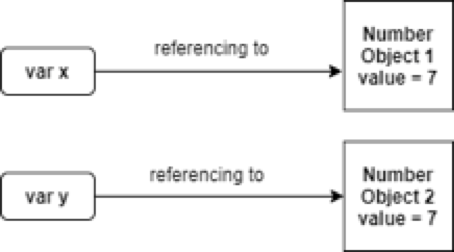# JavaScript: Difference between == and ===

June 13, 2020 251Today we are going to discuss the difference between == and === comparison operators in JavaScript. Both might work for certain comparisons but there can be times where misusage of them can lead to errors that might be difficult to debug. So, without further ado let’s get started!

## === Comparison Operator (Triple Equals)

The === operator is a strict comparison operator meaning that for both operands to be evaluated to true, the value and the type need to be the same.

## == Comparison Operator (Double Equals)

The == operator is an abstract (type-converting) comparison operator meaning that for both operands to be evaluated to true, only the contents need to be the same. What it does is it converts the values to the same type and then performs === comparison operation.

## Type-Conversion for == Operator

• The string value is converted to a number when comparing elements of type string and number.
• The Boolean value is converted to a number when comparing elements of type boolean and number i.e. false is converted to zero and true is converted to 1.
• The object is converted to a number when comparing elements of type object and number. If the object cannot be converted into a number, a runtime error is raised.
• The object is converted to a string when comparing elements of type object and string. If the object cannot be converted into a string, a runtime error is raised.

## Features of Comparison

• Two numbers are strictly equal if they have the same numeric value. For example, 3.3 is strictly equal to 3.3 but not to 3.33.
• Two strings are strictly equal if each of the corresponding characters is the same. For example, “good” is strictly equal to “good” but not to “goof” or “goods”.
• Two Boolean values are strictly equal if both are true or both are false.
• Two objects are strictly equal if they reference the same object.
• Positive zero and negative zero are strictly equal.
• Two objects with different contents are unequal for either abstract or strict comparisons.

The above-stated concepts will be clear by the examples below.

x = "codesdope"
y = "codesdope"
console.log(x==y)
console.log(x===y)


### Output

true true

The type and content of both the strings x and y are the same. Hence, the output == and === is also true. Remember that if the output of === is true, == is ultimately true as well and if the output of == is false, === will return false as well.

Let’s consider another example.

x = "5"
y = 5
console.log(x==5)
console.log(x===5)


### Output

true false

Here, the output of == operator is true and === operator is false. The type of x is string and y’s type is number and, thus, === returns false. What happens for the == operator is that it converts the string value to the number (type-conversion), then it performs the strict comparison. Now, the type and value are the same, hence, it is evaluated to be true.

Try to guess the result of the following code.

x = 0
y = false
console.log(x==y)
console.log(x===y)


### Output

true false

The same logic follows here i.e. x is of type number and y is of type boolean. Therefore, == returns true and === returns false.

Let’s see a few more examples before wrapping up.

NaN == NaN // false
NaN === NaN //false
null == undefined // true
null === undefined // false
null === null // true
"codesdope" != "blogsdope" // true
3.33 != "3.33" // false
3.33 !== "3.33" // true

x = new Number(7)
y = new Number(7)
x==y // false


The first six examples are straight-forward. The seven and eight examples contain the inverse of comparison operators meaning whatever the answer of the double equals operator or the triple equals operator is we just have to invert it.  The last one is a bit tricky. But, see that the type of x and y are the same, but both refer to different objects. Therefore, the answer is false. Note for two operands of the type object to be equal, they should reference the same object. This is illustrated in the figure below.That’s all you need to know about the two equality comparison operators. Use strict comparison when the type of operands needs to be the same.

Liked the post?
A computer science student having interest in web development. Well versed in Object Oriented Concepts, and its implementation in various projects. Strong grasp of various data structures and algorithms. Excellent problem solving skills.
0 COMMENT